# Simulation and Bayes Rule

In :
%matplotlib inline
import numpy as np
import pandas as pd
import matplotlib.pyplot as plt
import seaborn as sbn
from scipy.stats import norm, gamma, uniform
import pymc3 as pm3
from scipy import optimize
import corner as corner
import emcee as emcee

import warnings
warnings.filterwarnings('ignore')

sbn.set_style('white')
sbn.set_context('talk')

np.random.seed(12578)

/home/robhicks/anaconda/envs/python36/lib/python3.6/site-packages/h5py/__init__.py:36: FutureWarning: Conversion of the second argument of issubdtype from float to np.floating is deprecated. In future, it will be treated as np.float64 == np.dtype(float).type.
from ._conv import register_converters as _register_converters


Here, I'll briefly describe some of the step methods for producing a Monte Carlo Markov Chain. We have discussed in detail the random walk Metropolis Hastings sampler. We will briefly discuss in a bit more detail the following samplers:

1. Gibbs Samplers
2. Metropolis Hastings Samplers
a. Random Walk Metropolis Hastings
b. Adaptive Metropolis Hastings (we won't cover)
c. Independent Metropolis Hastings (we won't cover)
d. Slice Samplers and Other variants (we won't cover)

The nitty-gritty details of the following two samplers are beyond the scope of the course, but I will try to give you a feel for how they improve on Metropolis Hastings because they are known to be a major improvement over any of the samplers listed above even for high dimensional models (lots of coefficients to estimate):

• Hamiltonian Monte Carlo Sampler (No U-turn Sampler)
• Affine Invariant Sampler (EMCEE)

This is a very fast moving topic- so fast, in fact- that the current (non-beta) version of pymc doesn't have either NUTS or the Affine Invariant Sampler.

### Gibbs Sampler¶

The Gibbs Sampler is a special case of the Random-Walk Metropolis Hastings algorithm and one worth knowing about in a bit of detail because many tutorials and discussions about MH (especially older ones) are entertwined with discussions on Gibbs sampling and it can be confusing for the uninitiated. Despite this being a special case of MH, it is worth seeing how it works in a bit of detail. The idea is to successively sample each $\theta$ from its conditional distribution:

\begin{align} \theta^{t+1}_1 \sim & f_1(\theta^{t+1}_1 \mid \theta^{t}_2,\theta^{t}_3,\ldots, \theta^{t}_k,\mathbf{y},\mathbf{x}) \\ \theta^{t+1}_2 \sim & f_2(\theta^{t+1}_2 \mid \theta^{t+1}_1,\theta^{t}_3,\ldots, \theta^{t}_k,\mathbf{y},\mathbf{x}) \\ \theta^{t+1}_3 \sim & f_3(\theta^{t+1}_3 \mid \theta^{t+1}_1,\theta^{t+1}_2,\ldots, \theta^{t}_k,\mathbf{y},\mathbf{x})\\ &\vdots \\ \theta^{t+1}_k \sim & f_k(\theta^{t+1}_k \mid \theta^{t+1}_1,\theta^{t+1}_2,\theta^{t+1}_3\ldots, \theta^{t+1}_{k-1},\mathbf{y},\mathbf{x}) \end{align}

where each parameter $i$ has a conditional distribution function $f_i$.

First consider this simple example, where our parameters to be estimate are $\theta = [\mu,\sigma]$ and where we have flat priors:

In :
# data: construct toy data matrix
N = 1000
Y = norm(40,4).rvs(N)
plt.hist(Y);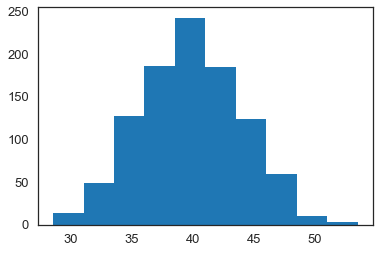When we employ a Gibbs Sampler, all samples are accepted (there are versions of the Gibbs Sampler that use a rejection based approach- not covered here). This is accomplished by choosing problems where the conditional distributions:

\begin{align} f_\mu(\mu | \sigma, \mathbf{Y}) \\ f_\sigma(\sigma | \mu, \mathbf{Y}) \end{align}

are known and have closed form solutions. For example, if we have

\begin{equation} f_\mu(\mu | \sigma, \mathbf{Y}) = \phi \left ( \frac{\mathbf{Y} - \mu}{\sigma} \right ) \end{equation}

that is, Y is distributed normal with mean $\mu$ and standard deviation $\sigma$, then it can be shown that \begin{equation} f_\sigma \left(\sigma^2 | \mu, \mathbf{Y}\right ) = InverseGamma(k,s) \end{equation}

where $k$ and $s$, the shape and scale parameters for the Inverse Gamma Distribution are \begin{align} k &= \frac{n}{2} \\ s &= \left (\frac{(Y-\mu)^2}{2} \right )^{-1} \end{align}

The Gibbs Sampler then proceeds as follows:

1. Start the sampler at an arbitrary starting value for $\theta$. In my code below, I start the Gibbs sampler at $\theta_0 = [\mu_0=30,\sigma_0=3]$.
2. Draw the next (always accepted) value of $\sigma_t$ by:
1. Draw random variate from a $\Gamma(k,s) = \Gamma \left ( \frac{n}{2},\left (\frac{(Y-\mu_{t-1})^2}{2} \right )^{-1} \right )$ and denote as $g$
2. Define $var_t = \frac{1}{g}$
3. Draw the next (always accepted) value of $\mu_t$ by:
1. Draw a normal random variate having mean $\bar{Y}$ and standard deviation $\sqrt{\frac{var_t}{n}}$ and denote as $\mu_t$.
4. Store the values $[\mu_t,\sqrt{var_t}]$ as the next sampled values for $\mu$ and $\sigma$.

1. Gibbs sampling depends on our ability to analytically solve for conditional distributions.
2. This involves derivations in mathematical statistics
3. Once we have these closed form solutions, we can accept every sampled draw
In :
chain_len = 10000
burnin = 1000

In :
# store chains here:
mu_chain_gibbs =np.zeros((chain_len+burnin,1))
sigma_chain_gibbs = np.zeros(mu_chain_gibbs.shape)

# starting guesses for mu
mu_old = 40

for i in np.arange(chain_len+burnin):
gamma_new = gamma(len(Y)/2,scale=1/np.sum(((Y-mu_old)**2)/2)).rvs()
var_new = 1/gamma_new
mu_new = norm(np.mean(Y), np.sqrt(var_new/len(Y))).rvs(1)
mu_chain_gibbs[i] = mu_new
sigma_chain_gibbs[i] = np.sqrt(var_new)
# reset old values
mu_old,var_old = mu_new,var_new

In :
# convert to pandas dataframe
chain_gibbs = pd.DataFrame(np.append(mu_chain_gibbs,sigma_chain_gibbs,1),columns=['mean','std'])
# calculate summary statistics post burn-in
chain_gibbs.loc[burnin:].describe(percentiles=[.025,.5,.975])

Out:
mean std
count 10000.000000 10000.000000
mean 39.805775 4.098301
std 0.129122 0.091833
min 39.305744 3.778357
2.5% 39.557243 3.927042
50% 39.805870 4.096343
97.5% 40.058110 4.286209
max 40.273004 4.507727
In :
plot_gibbs = chain_gibbs.loc[burnin:]
sbn.jointplot(x='mean', y='std', data=plot_gibbs, kind="kde");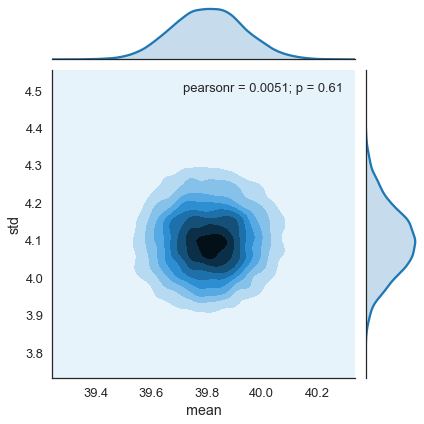In :
plt.figure(figsize=(12,5))
plt.plot(np.arange(chain_len),plot_gibbs['mean'])
plt.show()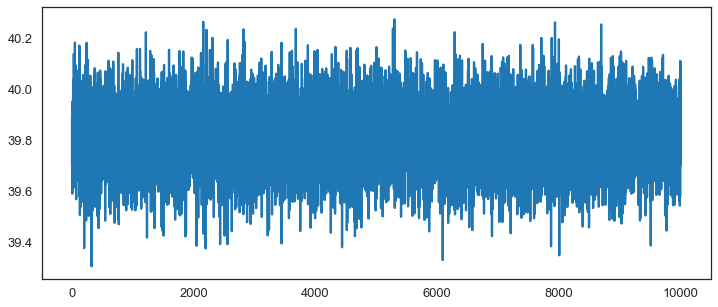The Gibbs Sampler was the predominant sampling method early on in applied Bayesian statistics because it was the empirical analogue of conjugate priors (the focus of Bayesian Statistics before the computer age) and does have real advantages over MH Random Walk for problems having solvable conditional distributions, since it accepts every sample and can be more efficient (less computing time to reach convergence and inference).

Despite this, both the Gibbs Sampler and the MH Sampler are less than ideal for

1. High dimensional problems (many parameters)
2. High correlation amongst parameters

### Bayesian OLS Regression applied to Tobias and Koop¶

We now turn our attention back to MH Random Walk sampling using a real dataset.

In :
tobias_koop=pd.read_csv('https://rlhick.people.wm.edu/econ407/data/tobias_koop_t_4.csv')
tobias_koop['ones'] = 1

Out:
id educ ln_wage pexp time ability meduc feduc broken_home siblings pexp2 ones
0 4 12 2.14 2 4 0.26 12 10 1 4 4 1
1 6 15 1.91 4 4 0.44 12 16 0 2 16 1
2 8 13 2.32 8 4 0.51 12 15 1 2 64 1
3 11 14 1.64 1 4 1.82 16 17 1 2 1 1
4 12 13 2.16 6 4 -1.30 13 12 0 5 36 1
In :
# sanity check on data:
from statsmodels.regression.linear_model import OLS
ols_res = OLS(tobias_koop.ln_wage,tobias_koop[['educ','pexp','pexp2','broken_home','ones']]).fit()
ols_res.summary()

Out:
Dep. Variable: R-squared: ln_wage 0.166 OLS 0.163 Least Squares 51.36 Mon, 12 Mar 2018 1.83e-39 13:02:26 -583.66 1034 1177. 1029 1202. 4 nonrobust
coef std err t P>|t| [0.025 0.975] 0.0853 0.009 9.179 0.000 0.067 0.104 0.2035 0.024 8.629 0.000 0.157 0.250 -0.0124 0.002 -5.438 0.000 -0.017 -0.008 -0.0087 0.036 -0.244 0.807 -0.079 0.061 0.4603 0.137 3.353 0.001 0.191 0.730
 Omnibus: Durbin-Watson: 55.892 1.761 0 112.05 -0.355 4.66e-25 4.448 391

This is the model statement describing priors and the likelihood. Here, we have 4 beta variables and sigma defines stochastic variables (we want a chain of sampled values for all of these variables) and we provide a prior distribution and hyper-parameters for each variable. The likelihood function is chosen to be Normal, having mean denoted as mu (which is defined as $\mathbf{x}\beta$, having standard deviation $\sigma$ (denoted as sigma). Our "dependent variable" is given by observed=tobias_koop.ln_wage, where tobias_koop.ln_wage is the log of wage from the Tobias and Koop dataset loaded above. In more formal terms, the code below sets up a basic_model having the following form:

\begin{align} Prob(\mu|\sigma,\mathbf{data}) \propto& Prob(\mathbf{data}|\mu,\sigma) \times Prob(\sigma | \mu^0_\sigma, \sigma^0_\sigma) \times Prob(\text{prior on }\beta) \\ Prob(\mu|\sigma,\mathbf{data}) \propto& \phi \left( \frac{\mathbf{ln\_wage} - \mathbf{x}\beta}{\sigma} \right ) \times HalfNormal \left(\frac{\sigma - \mu^0_\sigma}{\sigma^0_\mu} \right ) \times C \\ Prob(\mu|\sigma,\mathbf{data}) \propto& \phi \left( \frac{\mathbf{ln\_wage} - \mathbf{x}\beta}{\sigma} \right ) \times HalfNormal \left(\frac{\mu - 0}{10} \right ) \end{align}

where $\phi(.)$ is the standard normal pdf. The constant C (the joint probability of the prior on our $\beta$'s) is invariant with changes in our chain, and can therefore be dropped.

In :
ols_model = pm3.Model()

with ols_model:

# Priors for unknown model parameters
beta_0 = pm3.Flat('Intercept')
beta_1 = pm3.Flat('Educ')
beta_2 = pm3.Flat('Pexp')
beta_3 = pm3.Flat('Pexp2')
beta_4 = pm3.Flat('Broken Home')
sigma = pm3.HalfNormal('sigma', sd=10)

mu = beta_0 + beta_1*tobias_koop.educ + beta_2*tobias_koop.pexp + \
beta_3*tobias_koop.pexp2 + beta_4*tobias_koop.broken_home

# Likelihood (sampling distribution) of observations
ln_wage = pm3.Normal('ln_wage', mu=mu, sd=sigma, observed=tobias_koop.ln_wage)


### Metropolis-Hastings Random Walk¶

In :
chain_length = 20000

with ols_model:

start = pm3.find_MAP()
print(start)

# instantiate sampler
step = pm3.Metropolis()

# draw posterior samples
trace_mh = pm3.sample(chain_length, step=step, start=start, njobs=3, progressbar=True)

logp = -586.21, ||grad|| = 44.921: 100%|██████████| 76/76 [00:00<00:00, 1199.78it/s]

{'Intercept': array(0.46033436), 'Educ': array(0.08527245), 'Pexp': array(0.20352106), 'Pexp2': array(-0.01241256), 'Broken Home': array(-0.00872437), 'sigma_log__': array(-0.85446739), 'sigma': array(0.42550976)}

Multiprocess sampling (3 chains in 3 jobs)
CompoundStep
>Metropolis: [sigma_log__]
>Metropolis: [Broken Home]
>Metropolis: [Pexp2]
>Metropolis: [Pexp]
>Metropolis: [Educ]
>Metropolis: [Intercept]
100%|██████████| 20500/20500 [00:42<00:00, 481.22it/s]
The estimated number of effective samples is smaller than 200 for some parameters.

In :
pm3.traceplot(trace_mh[10000:]);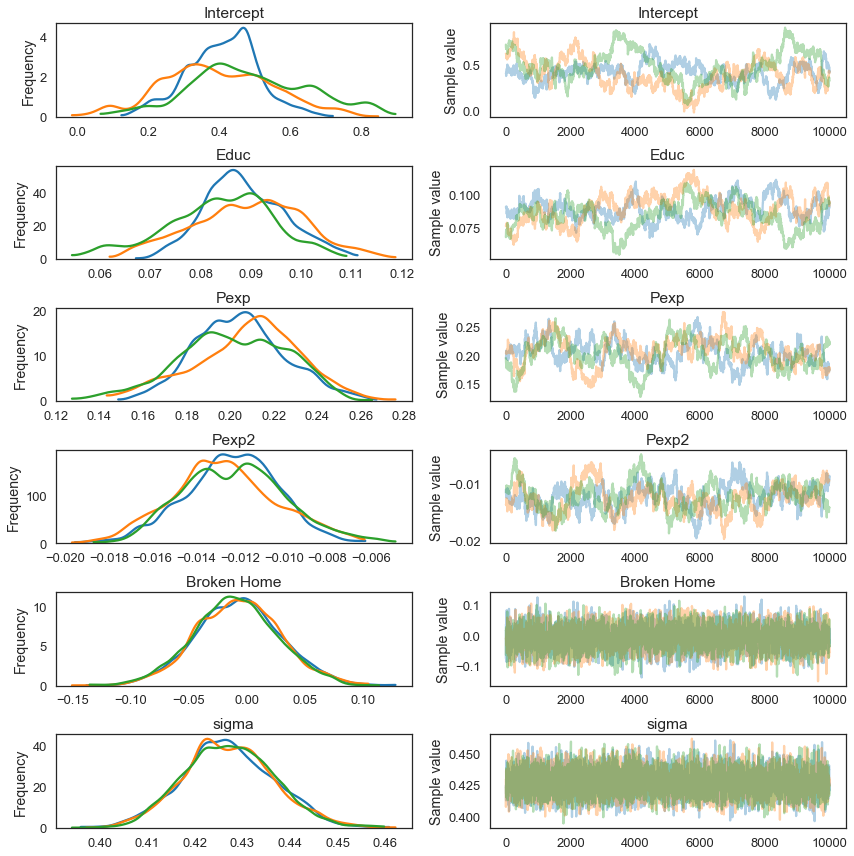In :
pm3.summary(trace_mh[10000:])

Out:
mean sd mc_error hpd_2.5 hpd_97.5 n_eff Rhat
Intercept 0.430862 0.148007 0.013392 0.155816 0.754521 26.0 1.057645
Educ 0.087346 0.010274 0.000905 0.066466 0.107397 28.0 1.050141
Pexp 0.204444 0.023515 0.002011 0.157057 0.248346 60.0 1.010773
Pexp2 -0.012436 0.002301 0.000192 -0.016984 -0.008021 67.0 1.005912
Broken Home -0.007875 0.036090 0.000689 -0.079255 0.064293 2604.0 1.001852
sigma 0.427079 0.009382 0.000130 0.409690 0.445975 5985.0 0.999971
In :
pm3.autocorrplot(trace_mh[2000:]);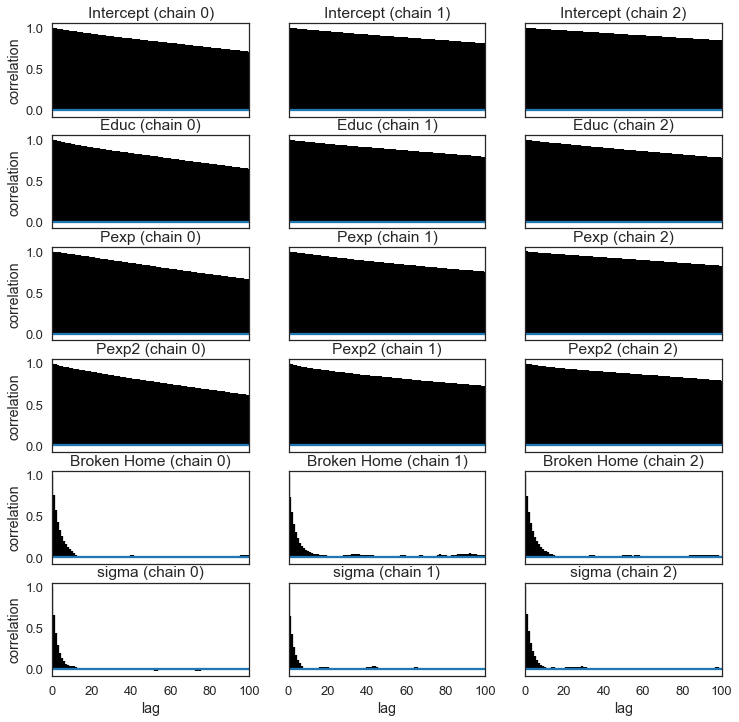In :
pm3.gelman_rubin(trace_mh[10000:])

Out:
{'Broken Home': 1.0018521528534778,
'Educ': 1.0501406764673435,
'Intercept': 1.0576453143671942,
'Pexp': 1.0107734289501882,
'Pexp2': 1.005912286669081,
'sigma': 0.9999711245444545}
In :
scores = pm3.geweke(trace_mh[10000:])

In :
# create plot for each variable:
varnames = list(scores)
varnames.remove('sigma_log__') #sigma_log__ is nuisance variable, ignore
chainids = scores.keys()
colors_for_chains = ['k','g','b']

plt.figure(figsize=(10,15))
plt.suptitle('Geweke Plot',fontsize=25)

plotnum = 1
for i in varnames:
plt.subplot(len(varnames),1,plotnum)
plt.title(i)
for j in chainids:
plt.scatter(scores[j][i][:,0],scores[j][i][:,1],s=50,c=colors_for_chains[j])

plt.axhline(-1.98, c='r')
plt.axhline(1.98, c='r')
plt.ylim(-2.5,2.5)
plt.xlim(-50,4050)
plotnum+=1

plt.tight_layout(rect=[0, 0.03, 1, 0.95])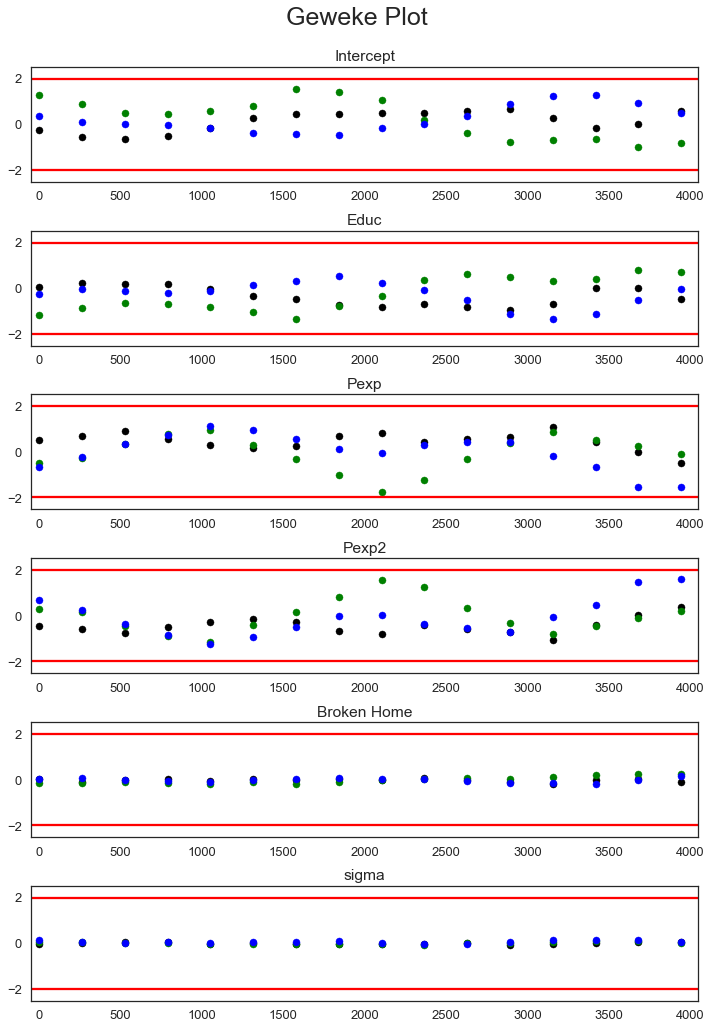I like visualizing my distributions using the corner plot function. To do that, convert your chains into a pandas dataframe:

In :
data = np.column_stack((trace_mh[10000:]['Intercept'],trace_mh[10000:]['Educ'],
trace_mh[10000:]['Pexp'],trace_mh[10000:]['Pexp2'],
trace_mh[10000:]['Broken Home'],trace_mh[10000:]['sigma']))
pairplot_dat = pd.DataFrame(data,columns=['Intercept','Educ','Pexp','Pexp2','Broken Home','sigma'])

In :
fig = corner.corner(pairplot_dat, labels=["Int", "$educ$", "$pexp$", "$pexp2$","$broken\_home$","sigma"],
truths=[.4603, .0853, .2035, -.0124, -.0087, np.sqrt(ols_res.mse_resid)])